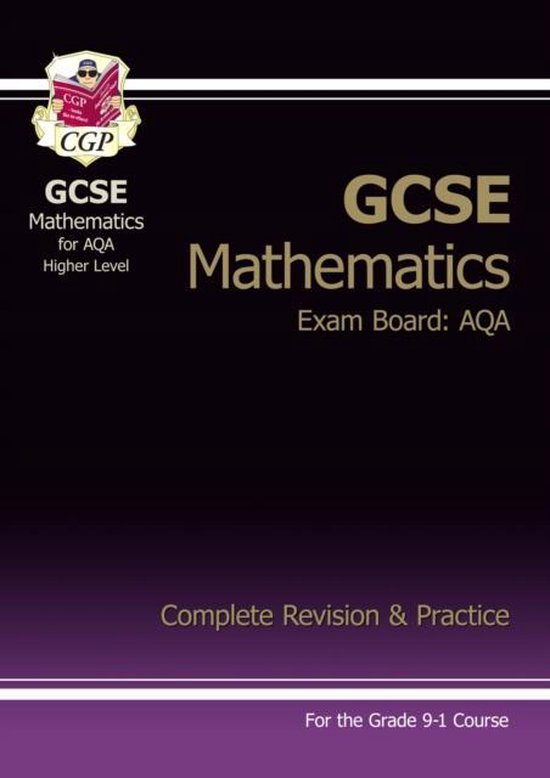• 16 Search Results 'aqa gcse maths'
• 0

Filter(s)

### All 16 resultsAQA GCSE Maths - formulae & equations flashcards
(0)
\$4.85

These flashcards are useful for learning and memorising key formulae & equations for your exams (across any exam board), with exam-style worked examples to support your understanding. (topics included: volume, surface area, sector area, arc length, quadratic formula)

•  Book
• Summary
•  • 8 pages •
• by justjosheela •
Quick View
i x
•
•AQA GCSE Maths - geometry & measures flashcards
(0)
\$4.14

These flashcards are useful in learning and memorising key topics within geometry & measures (across all exam boards), with diagrams to support your understanding. (topics included: angles in parallel lines, angles in polygons, special quadrilaterals)

•  Book
• Summary
•  • 5 pages •
• by justjosheela •
Quick View
i x
•
•AQA GCSE Maths - ratio & proportion flashcards
(0)
\$5.06

These flashcards are useful in learning and memorising key topics within ratio & proportion: each one shows the formula (both linear & triangles), with worked exam style examples. (topics included: indices & index laws, density, speed/distance/time, percentage change, pressure, simple interest.)

•  Book
• Summary
•  • 6 pages •
• by justjosheela •